Courses

# RD Sharma Solutions (Part - 2) - Ex-14.2, Lines and Angles, Class 7, Math Class 7 Notes | EduRev

## RD Sharma Solutions for Class 7 Mathematics

Created by: Abhishek Kapoor

## Class 7 : RD Sharma Solutions (Part - 2) - Ex-14.2, Lines and Angles, Class 7, Math Class 7 Notes | EduRev

The document RD Sharma Solutions (Part - 2) - Ex-14.2, Lines and Angles, Class 7, Math Class 7 Notes | EduRev is a part of the Class 7 Course RD Sharma Solutions for Class 7 Mathematics.
All you need of Class 7 at this link: Class 7

#### Question 13:

In Fig., if l || m || n and ∠1 = 60°, find ∠2.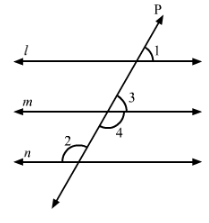In the given figure, l || m || and ∠1 = 60°.
Thus, we have:
∠3=∠1=60°     (Corresponding angle)
Now,
∠3+∠4=180°    (Linear pair)
∠4=180°−∠3=180°−60°=120°
∠2=∠4=120°      (Alternate interior angles)

Question 14:

In Fig., if AB || CD and CD || EF, find ∠ACE.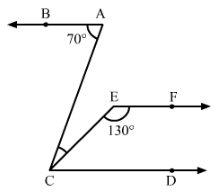In the given figure, AB || CD and CD || EF.
Extend line CE to E'.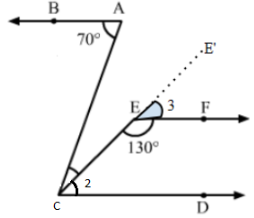Thus, we have:
∠BAC=∠ACD=70°              (Alternate angles)
Now,
∠3+∠CEF=180°                   (Linear pair)
⇒∠3=180°−∠CEF=180°−130°=50°

Since CD||EF, then
∠2=∠3=50°                (Corresponding angles)
∠ACE=∠ACD−∠2=70°−50°=20°

#### Question 15:

In Fig., if l || mn || p and ∠1 = 85°, find ∠2.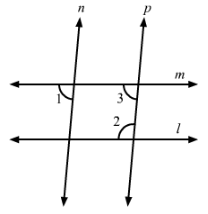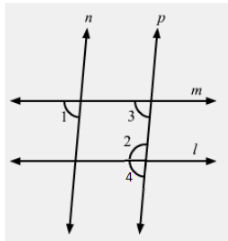In the given figure, l || mn || p and ∠1 = 85°.
Now, let ∠4 be the adjacent angle of ∠2.
Thus, we have:
∠3=∠1=85°        (Corresponding angles)

∠3+∠2=180°      (Sum of interior angles on the same side of the transversal)
∴∠2=180°−∠3=180°−85°=95°

#### Question 16:

In Fig., a transversal n cuts two lines l and m. If ∠1 = 70° and ∠7 = 80°, is l || m?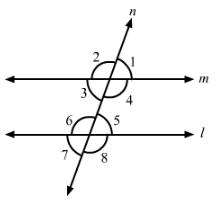We know that if the alternate exterior angles of two lines are equal, then the lines are parallel.
In the given figure, ∠1 and ∠7∠1 and ∠7 are alternate exterior angles, but they are not equal.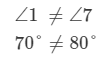Therefore, lines l and m are not parallel.

#### Question 17:

In Fig., a transversal n cuts two lines l and m such that ∠2 = 65° and ∠8 = 65°. Are the lines parallel?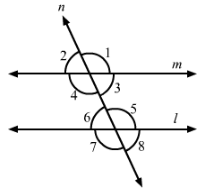∠2 = 3 = 65°        (Vertically opposite angles)
6 = 65°         (Vertically opposite angles)
∴ 3 = 6
⇒ l || m                       (Two lines are parallel if the alternate angles formed with the transversal are equal)

#### Question 18:

In Fig., show that AB || EF.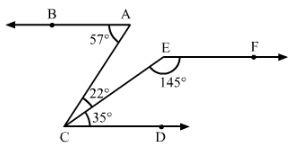Extend line CE to E'.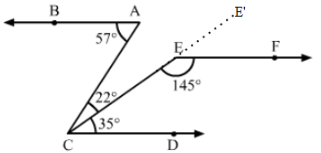∠BAC=57°=22°+35°=∠ACE+∠ECD
∴ AB||CD
Here, ∠E'EF+∠FEC=180°    (Linear pair)
⇒∠E'EF=180°−∠FEC=180°−145°=35°=∠ECD
∴EF||CD
Thus,
AB||CD ||EF

#### Question 19:

In Fig., AB || CD. Find the values of xyz.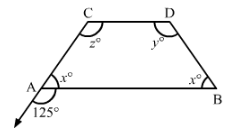∠x+125°=180°           (Linear pair)
∴∠x=180°−125°=55°

∠z=125°           (Corresponding angles)
∠x+∠z=180°   (Sum of adjacent interior angles is 180°180°)
∠x+125°=180°
⇒∠x=180°−125°=55°

∠x+∠y=180°          (Sum of adjacent interior angles is 180°180°)
55°+∠y=180°
⇒∠y=180°−55°=125°

#### Question 20:

In Fig., find out ∠PXR, if PQ || RS.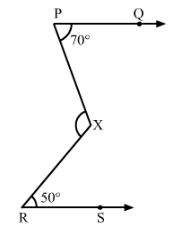Draw a line parallel to PQ passing through X.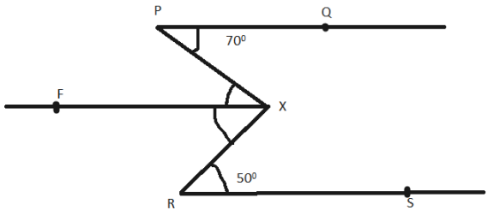Here,
∠PQX=∠PXF=70° and ∠SRX=∠RXF=50°      (Alternate interior angles)
∵ PQ || RS || XF
∴ ∠PXR=∠PXF+∠FXR=70°+50°=120°

#### Question 21:

In Fig., we have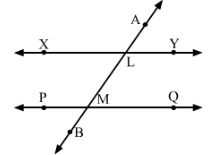(i) ∠MLY = 2 ∠LMQ, find ∠LMQ.
(ii) ∠XLM = (2x − 10)° and ∠LMQ = x + 30°, find x.
(iii) ∠XLM = ∠PML, find ∠ALY
(iv) ∠ALY = (2x − 15)°, and ∠LMQ = (x + 40)°, find x

(i)
∠LMQ=∠ALY          (Corresponding angles)
∴∠MLY+ ∠ALY=180°             (Linear pair)
⇒2∠ALY+∠ALY=180°
⇒3∠ALY=180°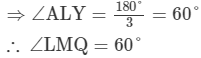(ii)
∠XLM=∠LMQ                (Alternate interior angles)
⇒(2x−10)°=(x+30)°
⇒2x−x=30°+10°
⇒x=40°

(iii)
∠ALX=∠LMP      (Corresponding angles)
∠ALX+∠XLM=180°         (Linear pair)
∠XLM=∠LMP         (Given)
∴∠LMP+∠LMP=180°
⇒2∠LMP=180°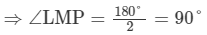XLM=∠LMP=90°
∠ALY=∠XLM       (Vertically opposite angles)
∴∠ALY=90°

(iv)
∠ALY=∠LMQ          (Corresponding angles)
∴(2x−15)°=(x+40)°
⇒2x−x=40°+15°
⇒x=55°

#### Question 22:

In Fig., DE || BC. Find the values of x and y.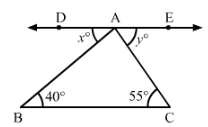∠ABC = ∠DAB       (Alternate interior angles)
∴ x=40°∴ x=40°

∠ACB = ∠EAC       (Alternate interior angles)
∴ y=55°

#### Question 23:

In Fig., line AC || line DE and ∠ABD = 32°. Find out the angles x and y if ∠E = 122°.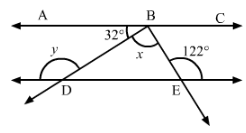∠BDE=∠ABD=32°            (Alternate interior angles)
⇒∠BDE+y=180°      (Linear pair)
⇒32°+y=180°
⇒y=180°−32°=148°

∠ABE=∠E=122°         (Alternate interior angle)
∠ABD+∠DBE=122°
32°+x=122°
x=122°−32°=90°

#### Question 24:

In Fig., side BC of ∆ABC has been produced to D and CE || BA. If ∠ABC = 65°, ∠BAC = 55°, find ∠ACE, ∠ECD and ∠ACD.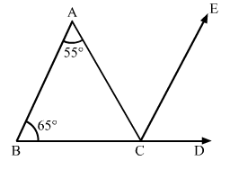∠ABC = ∠ECD = 55°          (Corresponding angles)
∠BAC = ∠ACE = 65°          (Alternate interior angles)
Now, ∠ACD = ∠ACE + ∠ECD
⇒ ∠ACD = 55° + 65° = 120°

#### Question 25:

In Fig., line CA ⊥ AB || line CR and line PR || line BD. Find ∠x, ∠y and ∠z.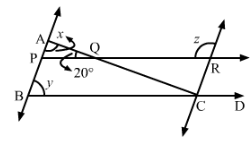Since CA ⊥ AB,
∴∠x=90°∴∠x=90°
We know that the sum of all the angles of triangle is 180°.
In ΔAPQ,
∠QAP+∠APQ+∠PQA=180°
⇒90°+∠APQ+20°=180°
⇒110°+∠APQ=180°
⇒∠APQ=180°−110°=70

∠PBC = ∠APQ = 70°           (Corresponding angles)
Since ∠PRC+∠z=180°           (Linear pair)
∠PRC+∠z=180°           Linear pair
∴∠z=180°−70°=110°    [∠APQ=∠PRC   (Alternate interior angles)]

#### Question 26:

In Fig., PQ || RS. Find the value of x.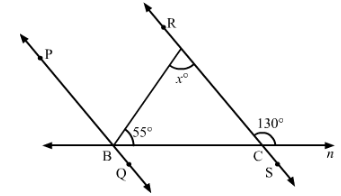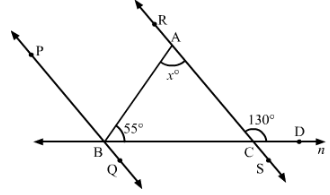∠RCD+∠RCB=180° (Linear pair)
⇒∠RCB=180°−130°=50°
In △ABC,
∠BAC+∠ABC+∠BCA=180°       (Angle sum property)
⇒∠BAC=180°−55°−50°=75°

#### Question 27:

In Fig., AB || CD and AE || CF; ∠FCG = 90° and ∠BAC = 120°. Find the values of xy and z.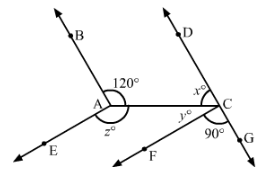∠BAC = ∠ACG = 120°          (Alternate interior angle)
∴ ∠ACF + ∠FCG = 120°
⇒ ∠ACF = 120° − 90° = 30°

∠DCA + ∠ACG = 180°            (Linear pair)
⇒∠x = 180° − 120° = 60°

∠BAC + ∠BAE + ∠EAC = 360°
∠CAE = 360° − 120° − (60° + 30°) = 150°             (∠BAE =  ∠DCF)

97 docs

,

,

,

,

,

,

,

,

,

,

,

,

,

,

,

,

,

,

,

,

,

,

,

,

,

,

,

,

,

,

;